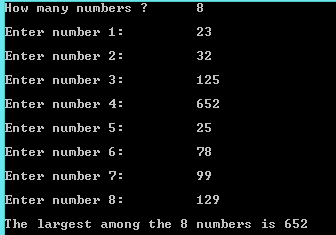## Thursday, 8 January 2015

### C Program to find the Largest Element in an Array

This is a C Program which finds the largest element in an array.

Here initially first element in array and  its next element are compared array and array , if array is greater then the value is stored in array and now the second element is increased 2 .3 4 .. until the last element is reached.

PROGRAM :
```#include <stdio.h>
#include <stdlib.h>

int main()
{
int i,n;
printf("How many numbers ?\t");
scanf("%d",&n);
int array[n];
for(i=0;i<n;i++)
{
printf("\nEnter number %d: \t",i+1);
scanf("%d",&array[i]);
}
for(i=0;i<n;i++)
{
if(array<array[i])
array=array[i];
}
printf("The largest among the %d numbers is %d",n,array);
return 0;
}
```
OUTPUT :C - Largest Element in an Array

Similar Programs :
C program to find the smallest element in an array
Cpp program to find the largest element in an array
Cpp program to find the smallest element in an array
Java program to find the largest  element in an array
Java program to find the smallest element in an array
Python program to find the largest and smallest element in a list